# Removing an element from a Binary Search Tree in Python

Posted on Mon 24 October 2022 in Python, Programming, Algorithms

💡 Note: Balancing the Binary Search Tree is out of scope for this blog post.

I’ve been taking Codecademy lessons recently and one of the bonus exercises was to figure out how to delete an element from a binary search tree. I figured I’d give it a shot.

``````class BinarySearchTree:
def __init__(self, value):
self.value = value
self.left = None
self.right = None
``````

Given a binary search tree, you can imagine our working tree to be this image below: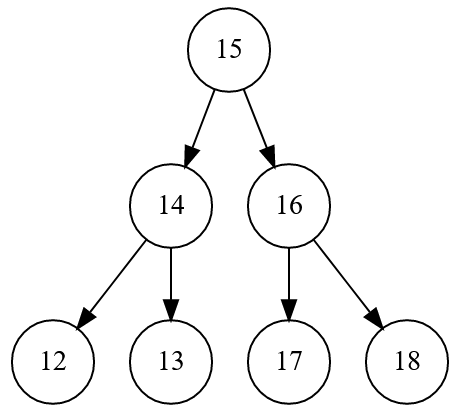# Example 1: Removing node 15, the parent node (Tree Rotation)

Removing 15 from the tree means that you’ll have two separate trees. To balance this all out, we can do a tree rotation.

First, we will get rid of 15 and make 16 the parent: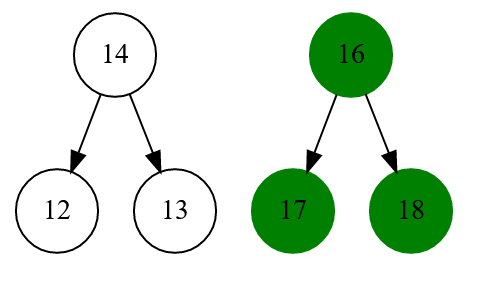Next, because the original left node (14) is orphaned, we would like to move 14 to be the leftmost node of the original tree (which would leave us with node 17).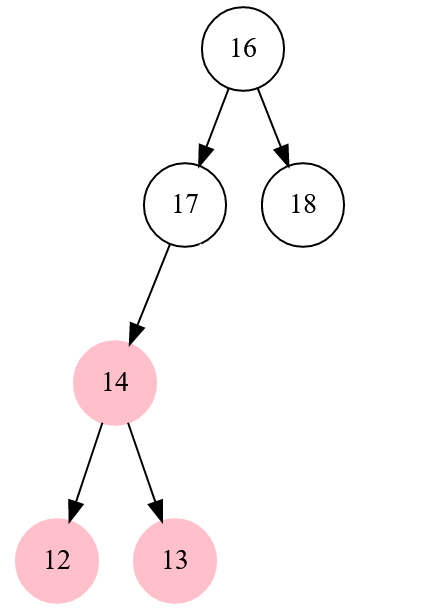We then add node 14 and its children to the previous leftmost node which is 17.

# Example 2: Removing node 18, a node without children

The work for this is straightforward. We remove Node 18 and we will not have to do any other operations. As you will see in the code below, 16 basically just de-references 18 and we’re good as gold.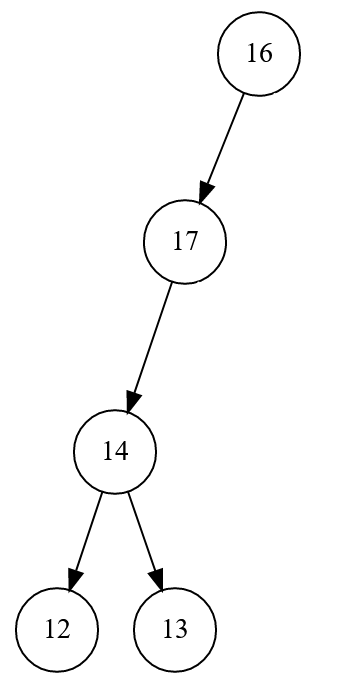# Example 3, Removing node 17 (Shift Left)

Removing 17 is pretty simple still because node 17 only has a left child. This means that we move node 14 to node 17’s position and not have to worry about an orphaned right node.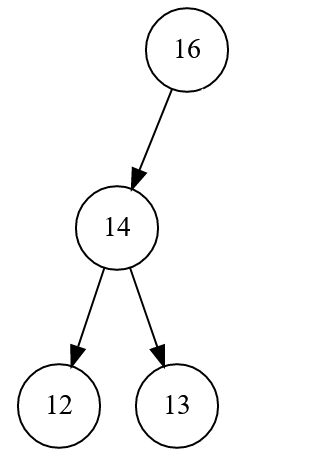# The Code

``````def remove_dft(self, value) -> bool:
if self.value == value:
if self.right:
# Move right node up to be the parent, then do tree rotation
self.right_rotation()
elif self.left:
# Move left node up to be the parent
self.left_shift()
else:
# No children, this node needs to be de-referenced
self.value = None
return True

if self.left:
found = self.left.remove_dft(value)
if found:
# Dereference empty node
if self.left.value == None:
self.left = None
return True

if self.right:
found = self.right.remove_dft(value)
if found:
# Dereference empty node
if self.right.value == None:
self.right = None
return True

return False

def left_shift(self):
if self.left:
# Shift left node to be the parent
self.value = self.left.value
self.right = self.left.right
self.left = self.left.left
return True

return False

def right_rotation(self):
if self.right:
# Rotate right node up
self.value = self.right.value
self.right = self.right.right

# Move orphaned left node into the leftmost node of the new parent
orphaned_left_node = self.left
if orphaned_left_node and self.right and self.right.left:
leftmost_node = self.right.left
while leftmost_node.left:
leftmost_node = leftmost_node.left
leftmost_node.left = orphaned_left_node
else:
self.left = orphaned_left_node

return True

return False
``````

# Runtime

Because the tree isn’t necessarily balanced, the worst case runtime would be O(N) or linear.